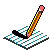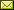PHYSICS@HORTONPhysics12

Physics 11

Math 11

NS Exams

Curriculum

Tips

Tutorials

McGraw Hill

Resources

Need it?

News

Enrichment

GiancoliIB Physics SL

April 2013 Notes

 April 2 Finish Error Analysis Lab Graphs of Motion review booklet April 3 April 4 BrainPop: Gravity What is Gravity? What is a Force? What Forces are Acting on You? Notes April 5 Investigating the Terminal Velocity of a Coffee Filter (DCP) April 8 Go over quiz Go over DCP lab Work on Terminal Velocity lab April 9 Work on Terminal Velocity lab Work through booklet (2.2 Forces & Dynamics)(p38-46) April 10 Forces & Dynamics (Resultant Forces/Balanced & Unbalanced Forces/Newton's First Law) Notes April 11 Forces & Dynamics (Translational Equilibrium/Newton's Second Law/Air Resitance) Forces & Motion: Basics (Tug of War & Acceleration Lab) (needs Java) Notes April 12 Forces & Dynamics (Newton's Second Law/Air Resitsance) Notes April 15 BrainPop: Force Finish Popper Physics Forces & Dynamics (Momentum & Newton's Second Law) Notes April 16 Forces & Dynamics (Impulse & Newton's Second Law/F-t graphs/Conservation of Linear Momentum) Momentum & Impulse More Momentum & Impulse Impulse Bouncing Ball Notes April 17 Forces & Dynamics (Conservation of Linear Momentum) pHET Collision Lab (needs JAVA!!! ~ settings: 1D, check velocity vectors & momenta diagram, try 100%, 50%, 0% elasticity, try different mass combinations, try different velocities and look at the total mometum before & after collision) Notes April 18 Forces & Dynamics (Conservation of Linear Momentum) Video: Momentum (Vector Addition) Notes April 19 Forces & Dynamics (Conservation of Linear Momentum) 2D Collisions (World-in-Motion video analysis) Another Example Notes April 23 Forces & Dynamics (Newton's Third Law) Notes April 24 Work, power, and energy (Forms of energy, conservation of energy, work) Notes April 25 Work, power, and energy (Work, force displacement graphs, Hooke's Law) Notes April 29 Work, power, and energy (Kinetic energy, gravitational potential energy, energy transformations) Energy Conservation in a Pendulum Energy Conservation ifn a Roller Coaster Notes April 30 QUIZ (Forces & dynamics) Work, power, and energy (Elastic Collisions) Notes

{subdirectory}